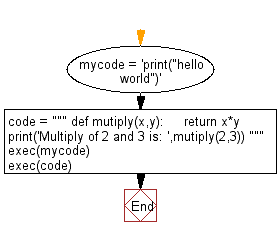﻿ Python Exercise: Execute a string containing Python code - w3resource# Python Exercise: Execute a string containing Python code

## Python Functions: Exercise - 18 with Solution

Write a Python program to execute a string containing Python code.

Sample Solution:-

Python Code:

``````mycode = 'print("hello world")'
code = """
def mutiply(x,y):
return x*y

print('Multiply of 2 and 3 is: ',mutiply(2,3))
"""
exec(mycode)
exec(code)
```
```

Sample Output:

```hello world
Multiply of 2 and 3 is:  6
```

Flowchart:Python Code Editor:

Have another way to solve this solution? Contribute your code (and comments) through Disqus.

What is the difficulty level of this exercise?

Test your Programming skills with w3resource's quiz.

﻿

## Python: Tips of the Day

Decapitalizes the first letter of a string:

Example:

```def tips_decapitalize(s, upper_rest=False):
return s[:1].lower() + (s[1:].upper() if upper_rest else s[1:])
print(tips_decapitalize('PythonTips'))
print(tips_decapitalize('PythonTips', True))
```

Output:

```pythonTips
pYTHONTIPS
```